These questions are based on the pie charts which represent the shift in water usage between 2006 and 2009; based on a survey conducted by Central Water Commission.
Water Usage in 2006 (In Trillion Litters )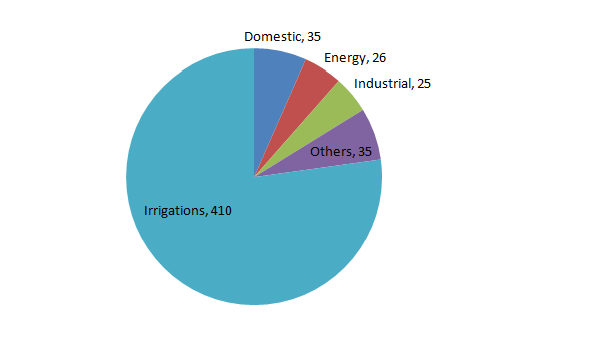Water Usage in 2009 (In Trillion Litters )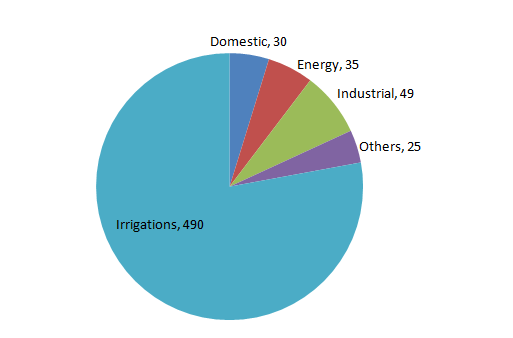Question 1: If the ratio of processing cost for water for industrial, energy and domestic usage is 3 : 5 : 2, what is the ratio of processing cost for above mentioned usage in 2006 to that in 2009?
(a) 0.68
(b) 0.72
(c) 0.60
(d) 0.77

Question 2: What is the percentage increase in usage in energy related sector from 2006 to 2009?
(a) 34.6%
(b) 23.3%
(c) 15.4%
(d) 17%

Question 3: What is the irrigation usage as percentage of total usage in 2009?
(a) 65.18%
(b) 83.45%
(c) 77.9%
(d) 69.6%

Question 4: What is the difference in domestic usage as percentage of total usage between 2006 and 2009?
(a) 3.16
(b) 2.08
(c) 2.26
(d) 1.91

Question 5: Which one out of the four shows the highest percentage increase in usage from 2006 to 2009?
(a) Domestic
(b) Industrial
(c) Others
(d) Energy

Ratio of cost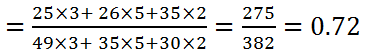The correct option is (b)

Required percentage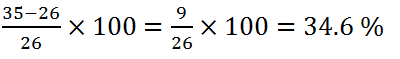The correct option is (a)

Required percentage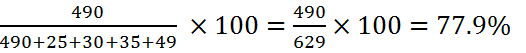The correct option is (c)

Required difference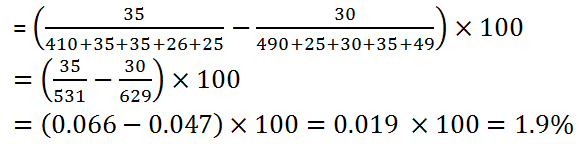The correct option is (d)